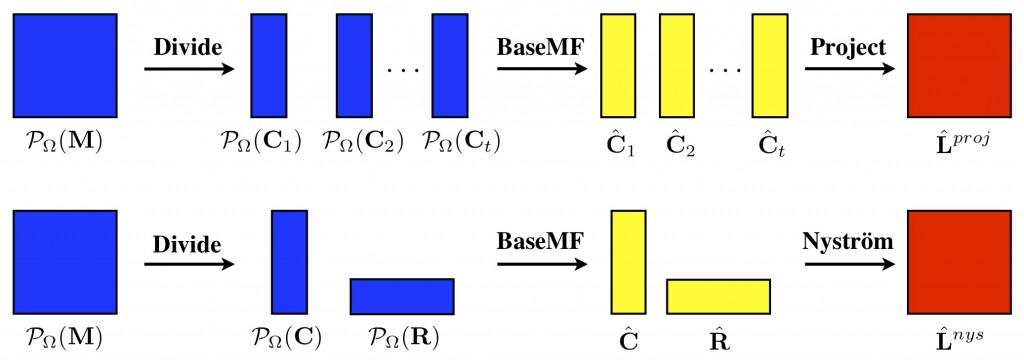# DFC — Divide-and-Conquer Matrix Factorization

Divide-Factor-Combine (DFC) is a parallel divide-and-conquer framework for noisy matrix factorization problems, e.g., matrix completion and robust matrix factorization. DFC divides a large-scale matrix factorization task into smaller subproblems, solves each subproblem in parallel using an arbitrary base matrix factorization algorithm, and combines the subproblem solutions using techniques from randomized matrix approximation.Our experiments with collaborative filtering, video background modeling, and simulated data demonstrate the near-linear to super-linear speed-ups attainable with this approach.  Moreover, our analysis shows that DFC enjoys high probability recovery guarantees comparable to those of its base algorithm.  More information about this work and an associated software package are available here